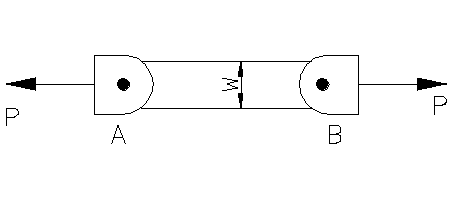# A 0.52 i n . -thick rectangular alloy bar is subjected to a tensile load P by pins at A and ...

## Question:

A {eq}0.52 \, in. {/eq}-thick rectangular alloy bar is subjected to a tensile load {eq}P {/eq} by pins at {eq}A {/eq} and {eq}B {/eq} as shown. The width of the bar is {eq}w = 3.2 \, in {/eq}. Strain gauges bonded to the specimen measure the following strains in the longitudinal {eq}(x) {/eq} and transverse {eq}(y) {/eq} directions: {eq}\varepsilon _{x}= 600 \, \mu \varepsilon {/eq} and {eq}\varepsilon {y} =-160 \mu \varepsilon {/eq}.

(a) Determine Poisson's ratio for this specimen.

(b) If the measured stains were produced by an axial load of {eq}P= 29 \, kips {/eq}, what is the modulus of elasticity for this specimen?## Modulus of Elasticity:

The modulus of elasticity is a ratio of stress acting on a given body and the strain produced in that body. The modulus of elasticity is valid up to an elastic point. It is defined for the axial stress. The unit of the elasticity is expressed in Newtons per square meter.

Given Data

• The thickness of the bar is: {eq}{t_{bar}} = 0.52\;{\rm{in}} {/eq}.
• The width of the bar is: {eq}w = 3.2\;{\rm{in}} {/eq}.
• The strain in...

Become a Study.com member to unlock this answer! Create your account